# RD Sharma Solutions For Class 7 Maths Exercise 16.2 Chapter 16 Congruence

RD Sharma Solutions for Class 7 Maths Exercise 16.2 of Chapter 16 Congruence in PDF are available here. Students can refer and download it from the available links. This exercise has ten main questions along with many sub-questions. RD Sharma Solutions for Class 7 provides solutions for all topics covered in this exercise. This exercise deals with the congruence of two triangles and sufficient condition for congruence of two triangles. Let us have a look at some of the important topics present in this exercise.

• Congruence of two triangles
• Sufficient condition for congruence of two triangles
• The side-side-side congruence condition

## Download the PDF of RD Sharma Solutions For Class 7 Chapter 16 – Congruence Exercise 16.2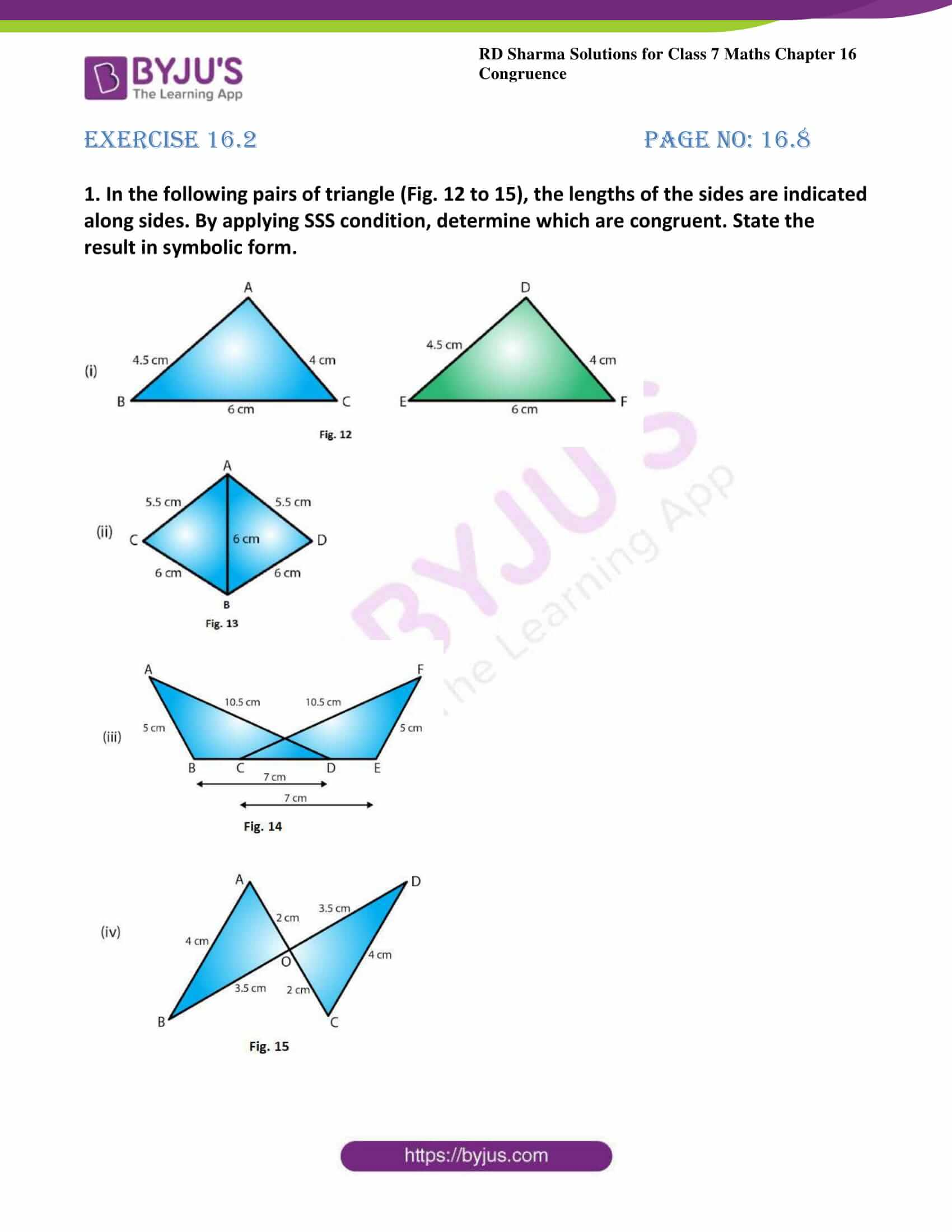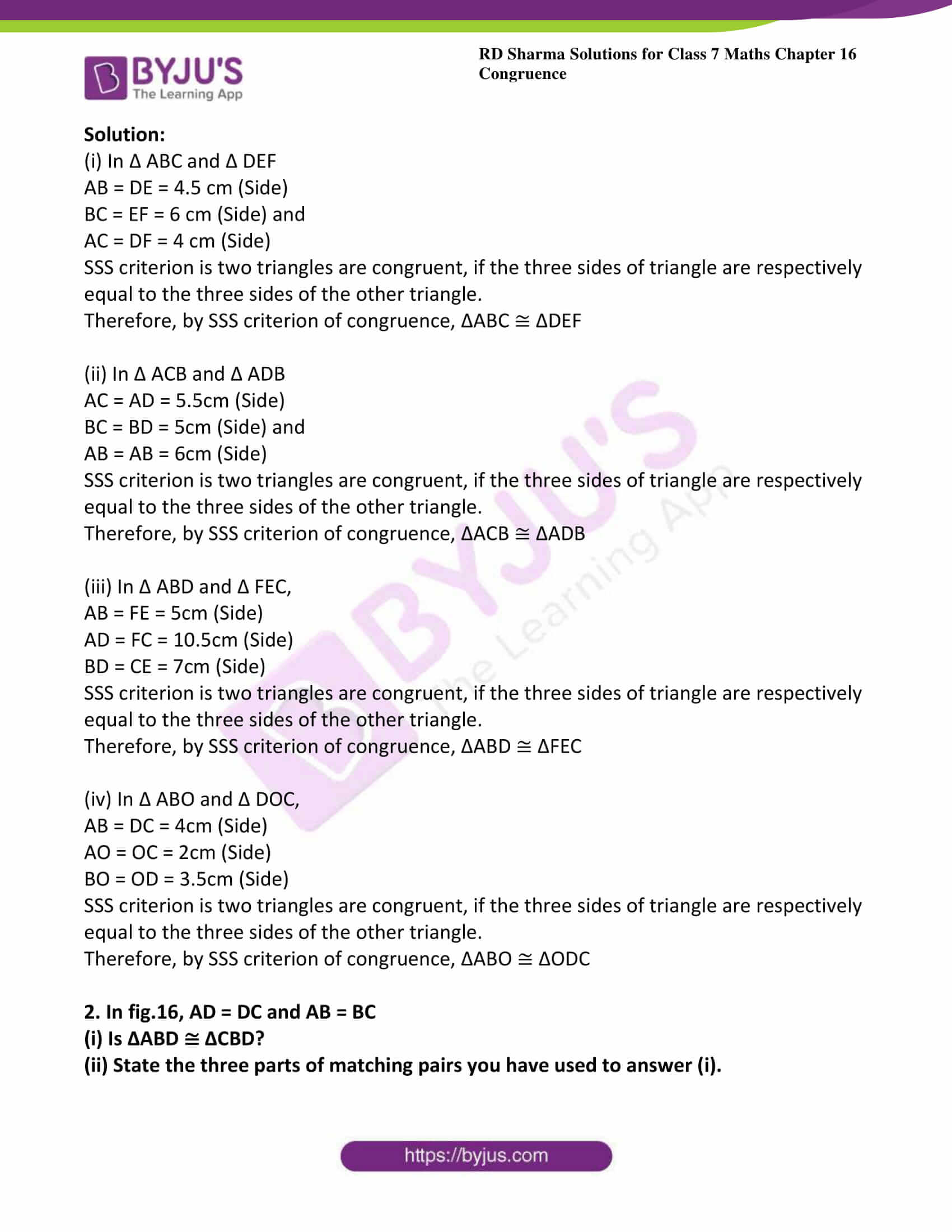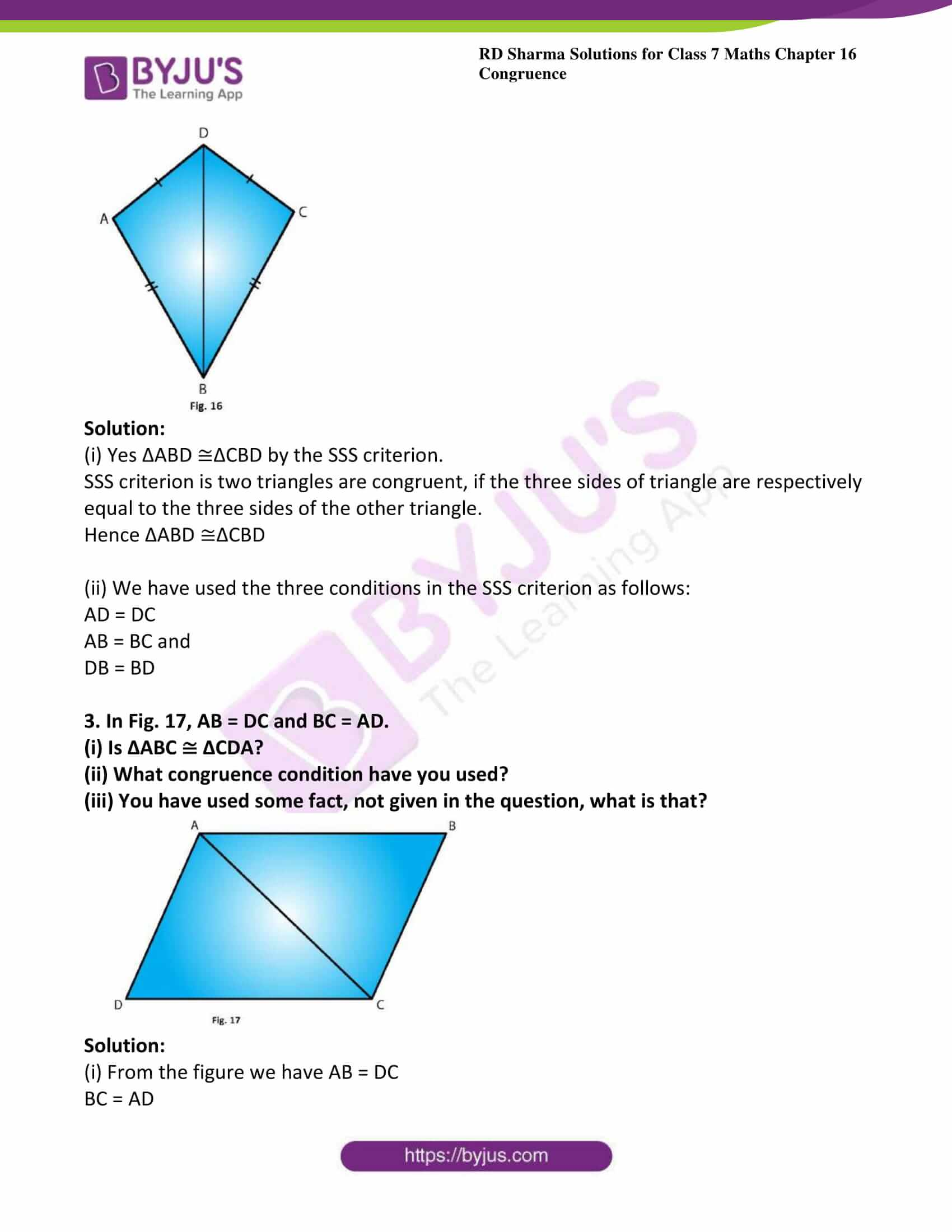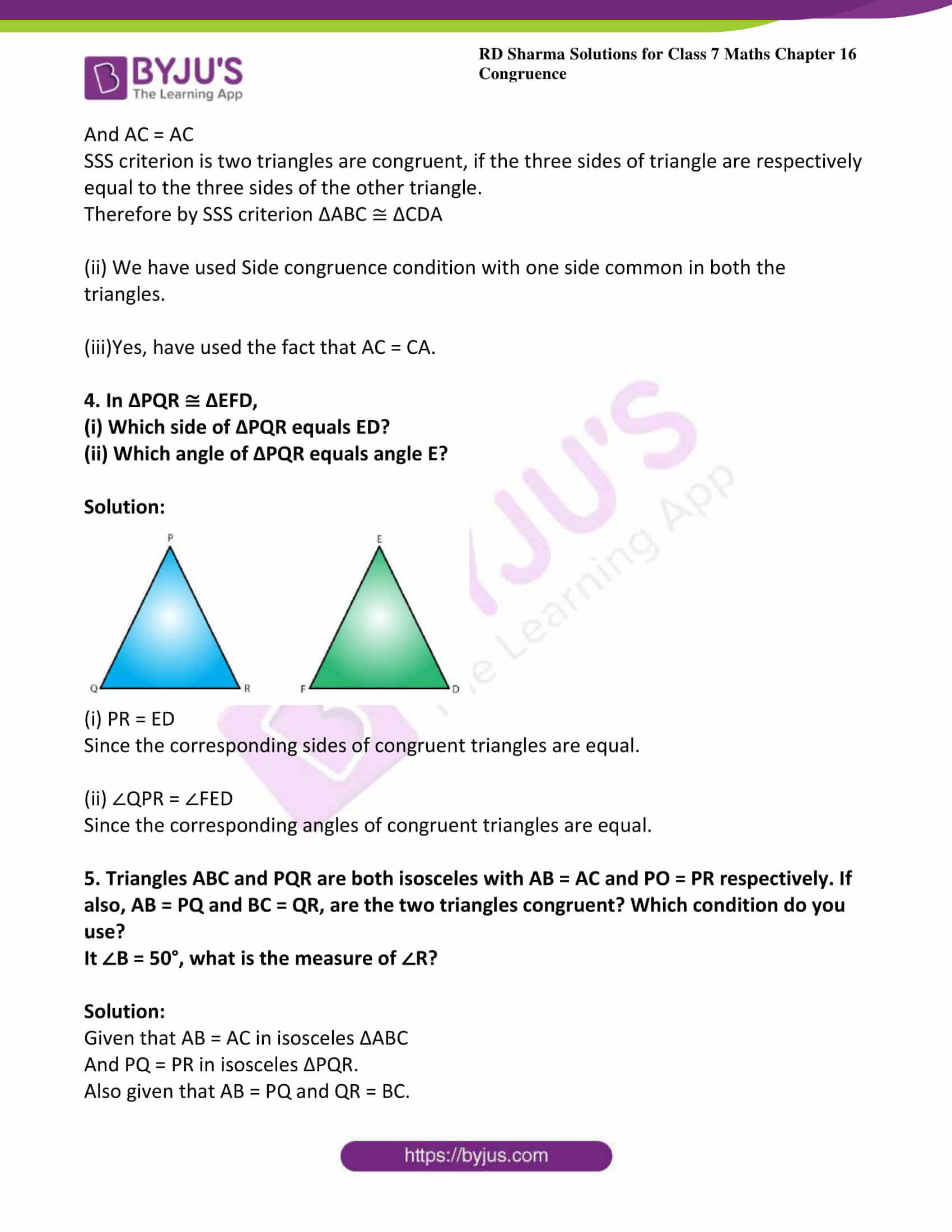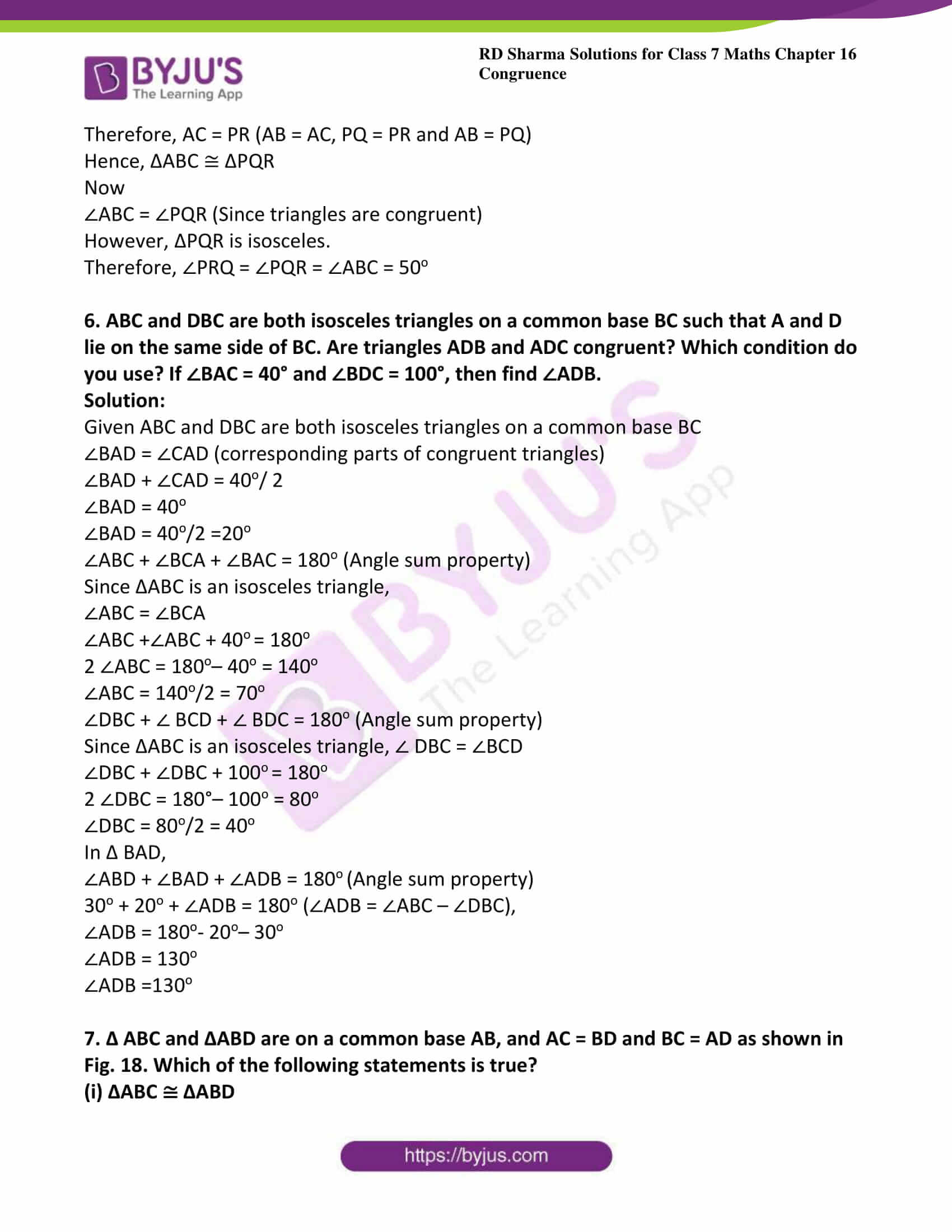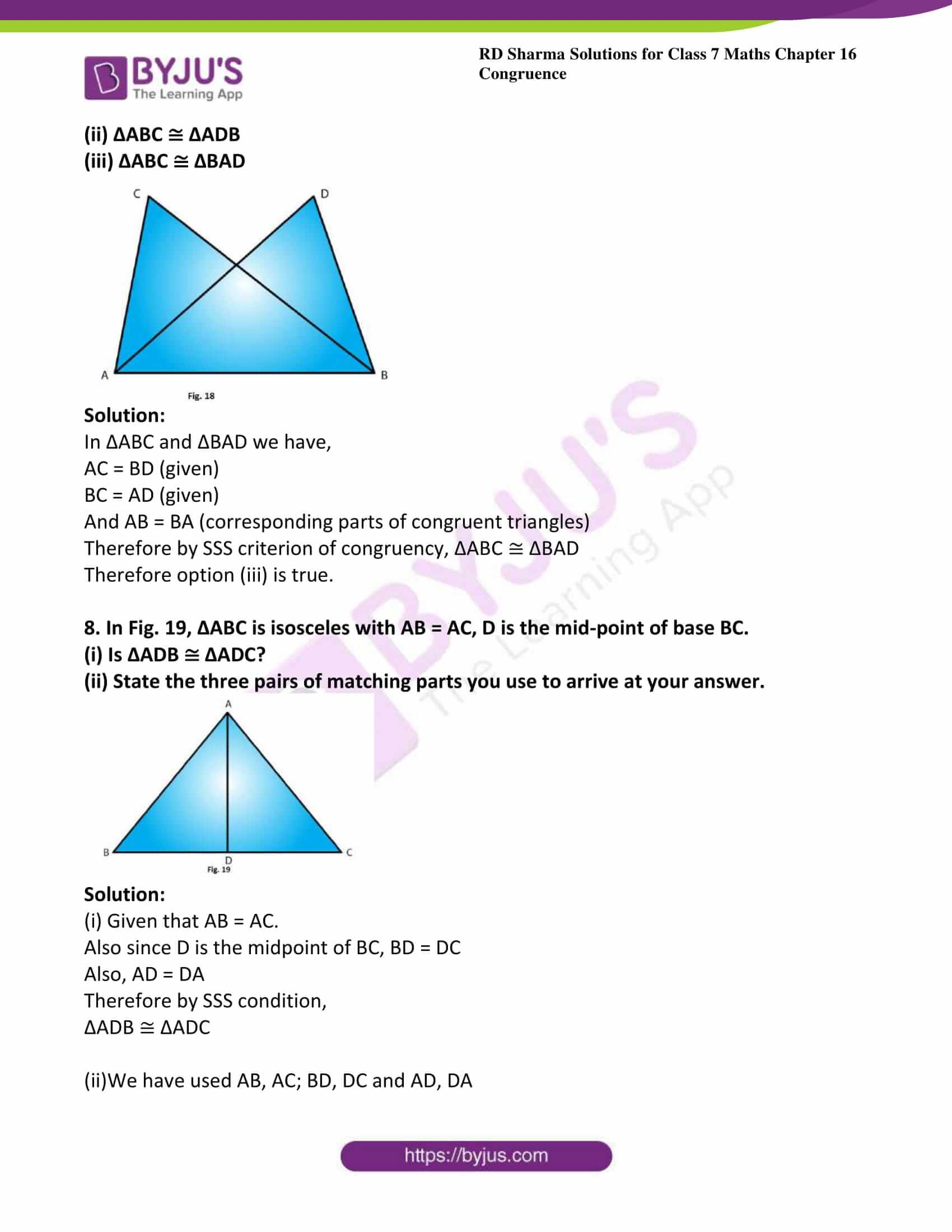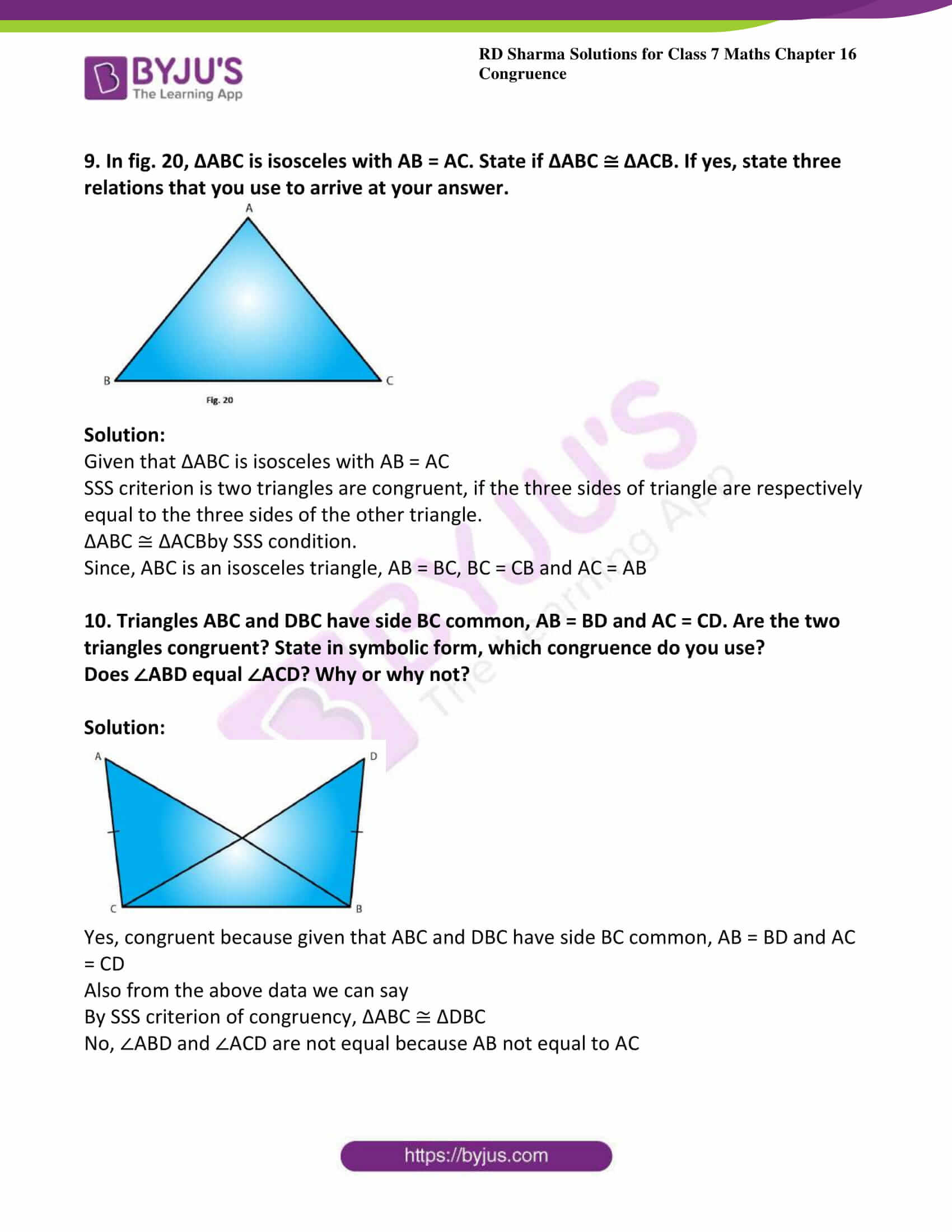### Access answers to Maths RD Sharma Solutions For Class 7 Chapter 16 – Congruence Exercise 16.2

1. In the following pairs of triangle (Fig. 12 to 15), the lengths of the sides are indicated along sides. By applying SSS condition, determine which are congruent. State the result in symbolic form.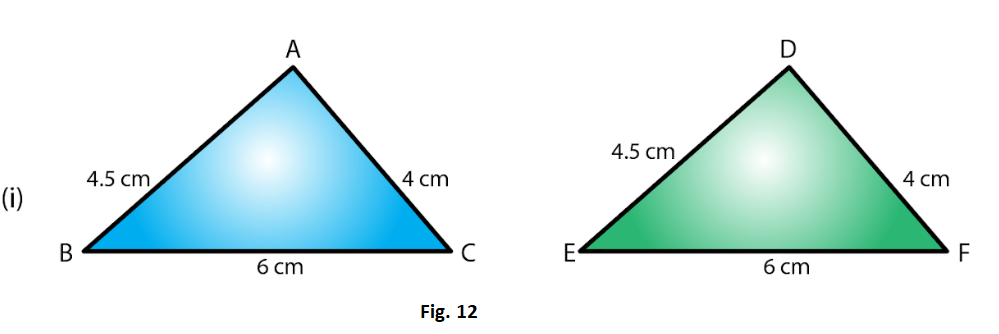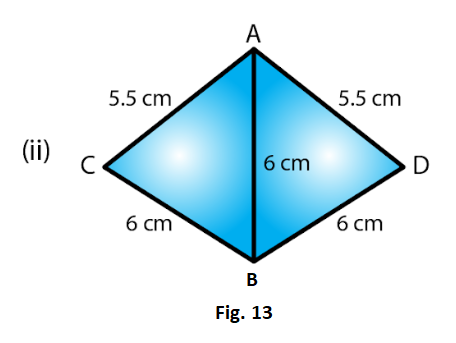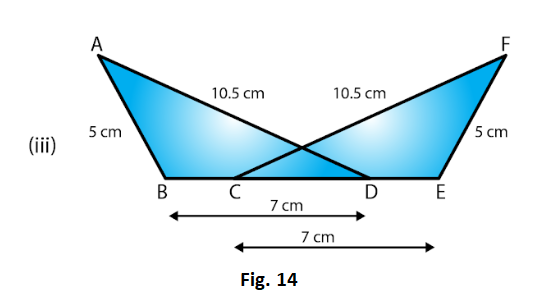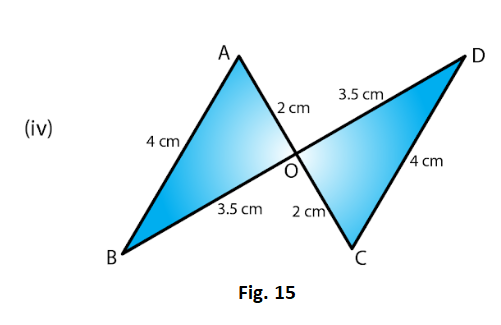Solution:

(i) In Δ ABC and Δ DEF

AB = DE = 4.5 cm (Side)

BC = EF = 6 cm (Side) and

AC = DF = 4 cm (Side)

SSS criterion is two triangles are congruent, if the three sides of triangle are respectively equal to the three sides of the other triangle.

Therefore, by SSS criterion of congruence, ΔABC ≅ ΔDEF

(ii) In Δ ACB and Δ ADB

AC = AD = 5.5cm (Side)

BC = BD = 5cm (Side) and

AB = AB = 6cm (Side)

SSS criterion is two triangles are congruent, if the three sides of triangle are respectively equal to the three sides of the other triangle.

Therefore, by SSS criterion of congruence, ΔACB ≅ ΔADB

(iii) In Δ ABD and Δ FEC,

AB = FE = 5cm (Side)

AD = FC = 10.5cm (Side)

BD = CE = 7cm (Side)

SSS criterion is two triangles are congruent, if the three sides of triangle are respectively equal to the three sides of the other triangle.

Therefore, by SSS criterion of congruence, ΔABD ≅ ΔFEC

(iv) In Δ ABO and Δ DOC,

AB = DC = 4cm (Side)

AO = OC = 2cm (Side)

BO = OD = 3.5cm (Side)

SSS criterion is two triangles are congruent, if the three sides of triangle are respectively equal to the three sides of the other triangle.

Therefore, by SSS criterion of congruence, ΔABO ≅ ΔODC

2. In fig.16, AD = DC and AB = BC

(i) Is ΔABD ≅ ΔCBD?

(ii) State the three parts of matching pairs you have used to answer (i).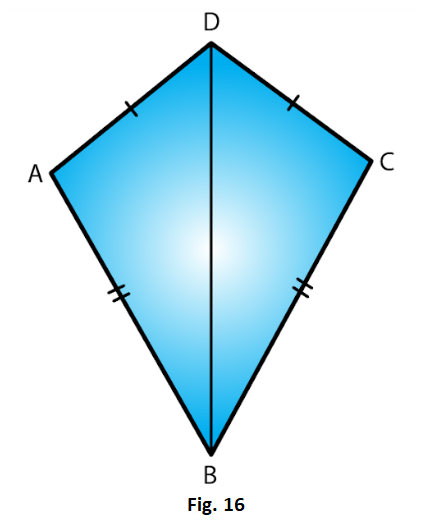Solution:

(i) Yes ΔABD ≅ΔCBD by the SSS criterion.

SSS criterion is two triangles are congruent, if the three sides of triangle are respectively equal to the three sides of the other triangle.

Hence ΔABD ≅ΔCBD

(ii) We have used the three conditions in the SSS criterion as follows:

AB = BC and

DB = BD

3. In Fig. 17, AB = DC and BC = AD.

(i) Is ΔABC ≅ ΔCDA?

(ii) What congruence condition have you used?

(iii) You have used some fact, not given in the question, what is that?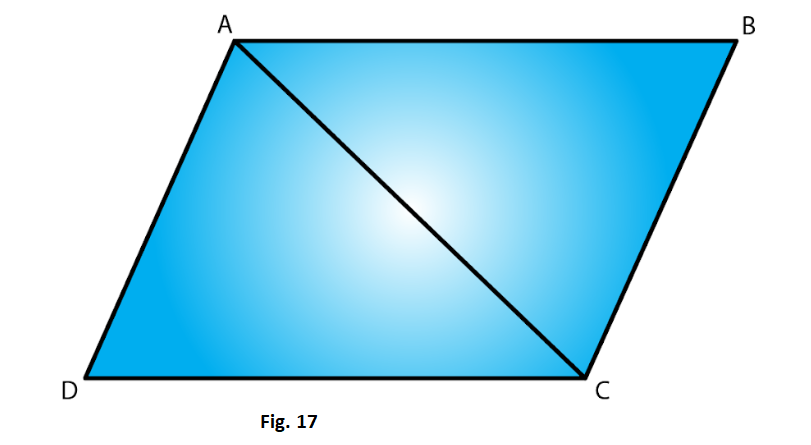Solution:

(i) From the figure we have AB = DC

And AC = AC

SSS criterion is two triangles are congruent, if the three sides of triangle are respectively equal to the three sides of the other triangle.

Therefore by SSS criterion ΔABC ≅ ΔCDA

(ii) We have used Side congruence condition with one side common in both the triangles.

(iii)Yes, have used the fact that AC = CA.

4. In ΔPQR ≅ ΔEFD,

(i) Which side of ΔPQR equals ED?

(ii) Which angle of ΔPQR equals angle E?

Solution: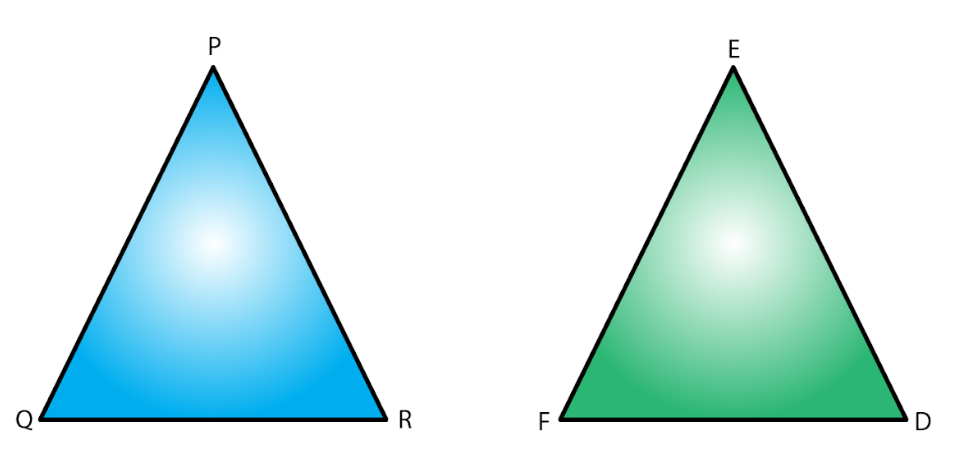(i) PR = ED

Since the corresponding sides of congruent triangles are equal.

(ii) ∠QPR = ∠FED

Since the corresponding angles of congruent triangles are equal.

5. Triangles ABC and PQR are both isosceles with AB = AC and PO = PR respectively. If also, AB = PQ and BC = QR, are the two triangles congruent? Which condition do you use?

It ∠B = 50°, what is the measure of ∠R?

Solution:

Given that AB = AC in isosceles ΔABC

And PQ = PR in isosceles ΔPQR.

Also given that AB = PQ and QR = BC.

Therefore, AC = PR (AB = AC, PQ = PR and AB = PQ)

Hence, ΔABC ≅ ΔPQR

Now

∠ABC = ∠PQR (Since triangles are congruent)

However, ΔPQR is isosceles.

Therefore, ∠PRQ = ∠PQR = ∠ABC = 50o

6. ABC and DBC are both isosceles triangles on a common base BC such that A and D lie on the same side of BC. Are triangles ADB and ADC congruent? Which condition do you use? If ∠BAC = 40° and ∠BDC = 100°, then find ∠ADB.

Solution:

Given ABC and DBC are both isosceles triangles on a common base BC

∠ABC + ∠BCA + ∠BAC = 180o (Angle sum property)

Since ΔABC is an isosceles triangle,

∠ABC = ∠BCA

∠ABC +∠ABC + 40o = 180o

2 ∠ABC = 180o– 40o = 140o

∠ABC = 140o/2 = 70o

∠DBC + ∠ BCD + ∠ BDC = 180o (Angle sum property)

Since ΔABC is an isosceles triangle, ∠ DBC = ∠BCD

∠DBC + ∠DBC + 100= 180o

2 ∠DBC = 180°– 100o = 80o

∠DBC = 80o/2 = 40o

30o + 20o + ∠ADB = 180o (∠ADB = ∠ABC – ∠DBC),

7. Δ ABC and ΔABD are on a common base AB, and AC = BD and BC = AD as shown in Fig. 18. Which of the following statements is true?

(i) ΔABC ≅ ΔABD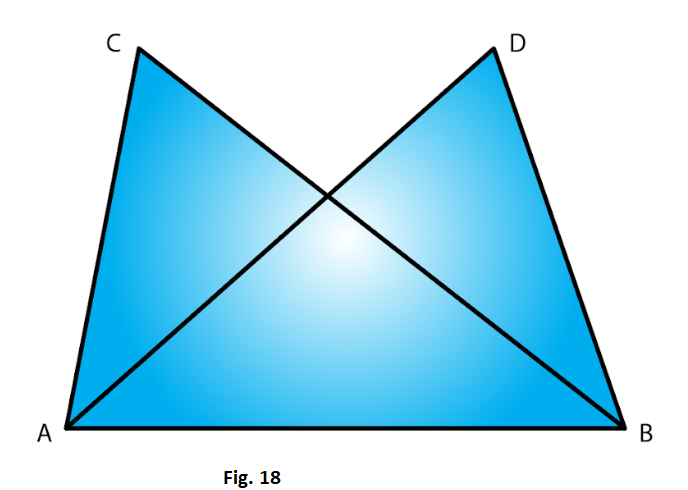Solution:

In ΔABC and ΔBAD we have,

AC = BD (given)

And AB = BA (corresponding parts of congruent triangles)

Therefore by SSS criterion of congruency, ΔABC ≅ ΔBAD

Therefore option (iii) is true.

8. In Fig. 19, ΔABC is isosceles with AB = AC, D is the mid-point of base BC.

(ii) State the three pairs of matching parts you use to arrive at your answer.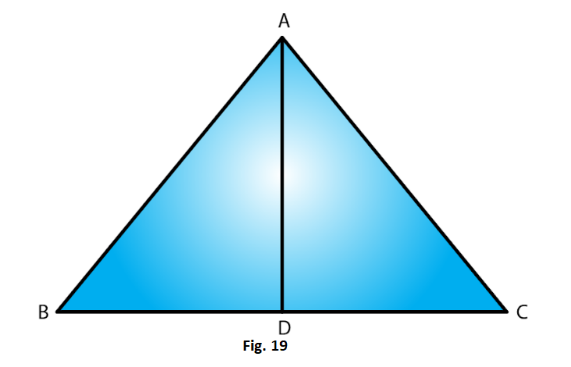Solution:

(i) Given that AB = AC.

Also since D is the midpoint of BC, BD = DC

Therefore by SSS condition,

(ii)We have used AB, AC; BD, DC and AD, DA

9. In fig. 20, ΔABC is isosceles with AB = AC. State if ΔABC ≅ ΔACB. If yes, state three relations that you use to arrive at your answer.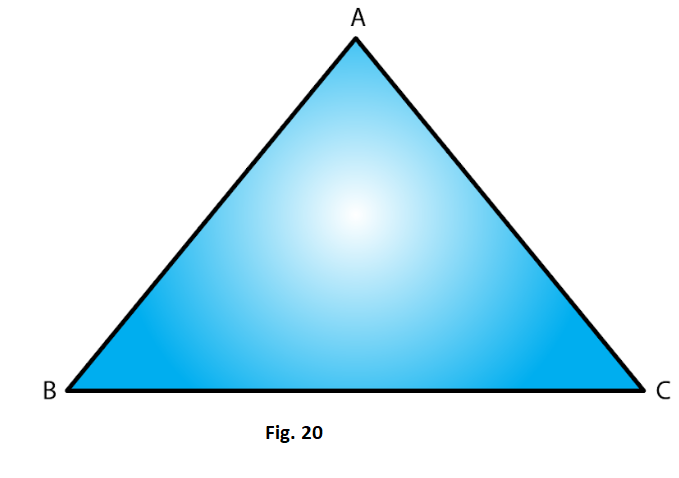Solution:

Given that ΔABC is isosceles with AB = AC

SSS criterion is two triangles are congruent, if the three sides of triangle are respectively equal to the three sides of the other triangle.

ΔABC ≅ ΔACBby SSS condition.

Since, ABC is an isosceles triangle, AB = BC, BC = CB and AC = AB

10. Triangles ABC and DBC have side BC common, AB = BD and AC = CD. Are the two triangles congruent? State in symbolic form, which congruence do you use? Does ∠ABD equal ∠ACD? Why or why not?

Solution: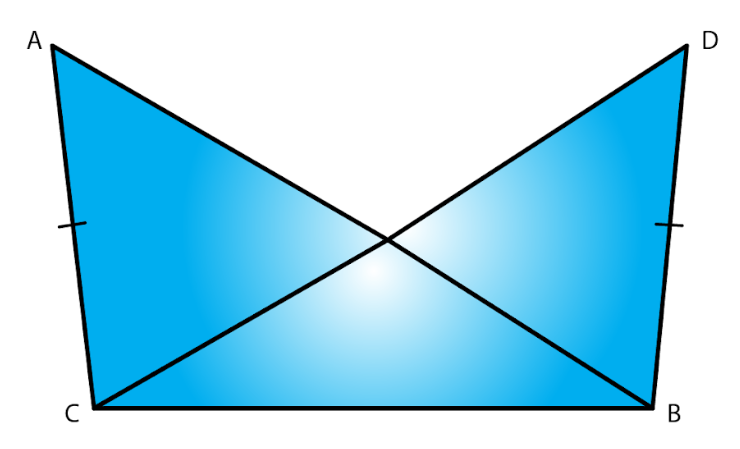Yes, congruent because given that ABC and DBC have side BC common, AB = BD and AC = CD

Also from the above data we can say

By SSS criterion of congruency, ΔABC ≅ ΔDBC

No, ∠ABD and ∠ACD are not equal because AB not equal to AC